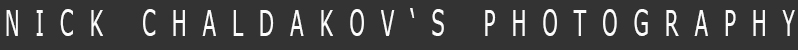c h a l d a k o v . c o m   s i n c e   1 9 9 9 W e l c o m е ! version 13.04 | site map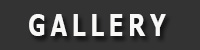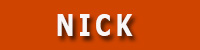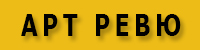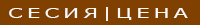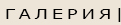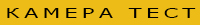N E W - A R T - P H O T O G R A P H Y ' 2 0 2 0
 Art photography - Nick Chaldakov
 A R T - P H O T O G R A P H Y - 1 9 9 1 - 2 0 1 2
 F I N E   A R T   P H O T O G R A P H Y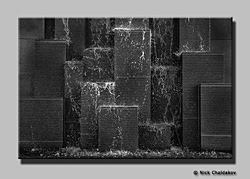S I M P L E   A B S T R A C T '1 9 9 1 - 0 1 N O   N A M E '2 0 0 3 D E S K T O P   P H O T O   S H O W '2 0 0 2 S P I R I T U A L   B L U E '2 0 0 5 V E N I C E   F R O M   B E L O W '2 0 0 5 T H E   T I M E '2 0 0 5
 B & W  P H O T O G R A P H Y
 X X X '2012 A N A T O M Y   O F   T I M E '2 0 1 0 M Y   C H A I R '2 0 0 7 A U T U M N   W O O D '2 0 0 6 Y O U   A N D   Y O U '2 0 0 6 O N   T H E   G R O U N D '20 0 4 O N   T H E   G R O U N D '2 0 0 4 T H E '  M E T A L '  W A T E R '2 0 0 2 M Y   K I T C H E N '2 0 0 1 M Y   S A N D A L '2 0 0 1 M Y   S T A I R '2 0 0 1 M Y   F A C E '2 0 0 1
 C O L O R   P H O T O G R A P H Y
 E R O T I C K '2009 T H E   N U T E '2 0 0 4 T H E   V I O L I N '2 0 0 6 T K - 8 0 ' 2 0 0 4 T H E   S A N D   T I M E '2 0 0 6 M I M '1 9 9 8 - 2 0 0 0
 С П И С А Н И Е   З А   Ф О Т О Г Р А Ф И Я   И   Н А Ч И Н   Н А   Ж И В О Т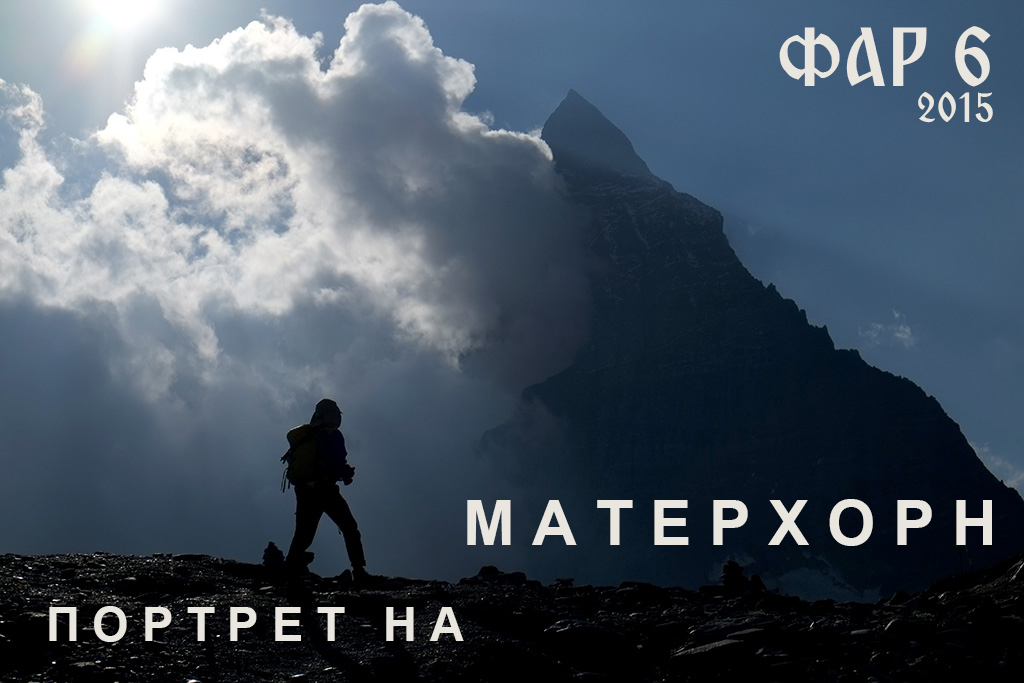C O N T A C T O N L I N E NICK CHALDAKOV

 www.chaldakov.com is a free photography gallery! In order to keep it this way please advertise at www.chaldakov.comY o u r   L i n k   H e r e | ADD URL